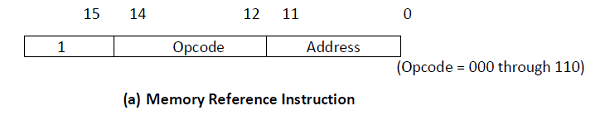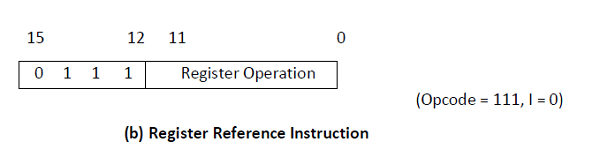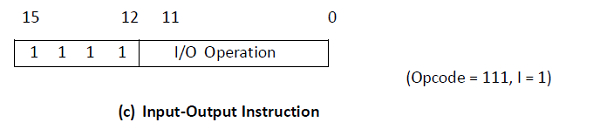# What are Computer Instructions?

A computer has programs stored in its RAM in the form of 1s and 0s that are interpreted by the CPU as instructions. One word of RAM includes one instruction in the machine language.

These instructions are loaded to the CPU one at a time, where it receives decoded and implemented. A basic computer has three instruction code formats such as the memory reference instruction, the register reference instruction, and the input-output instruction format.

## Memory Reference Instruction

A memory-reference instruction uses 12 bits to specify an address and one bit to determine the addressing mode I. I is the same as 0 for direct address and to 1 for indirect address.## Register Reference Instruction

The register reference instructions are identified by the operation code 111 with a 0 in the leftmost bit (bit 15) of the instruction. It determines an operation on or a test of the AC register. An operand from memory is not required because the additional 12 bits are used to determine the operation or test to be implemented.## Input-Output Instruction

An input-output instruction does not require a reference to memory and is identified by the operation code 111 with a 1 in the leftmost bit of the instruction. The remaining 12 bits can determine the type of input-output operation or test implemented.The type of instruction is identified by the computer control from the four bits in positions 12 through 15 of the instruction. If the three opcode bits in positions 12 through 14 are not similar to 111, the instruction is a memory-reference type and the bit in position 15 is taken as the addressing mode I.

If the 3-bit opcode is similar to 111, the control then examines the bit in position 15. If this bit is 0, the instruction is a register-reference type. If the bit is 1, the instruction is an input-output type.